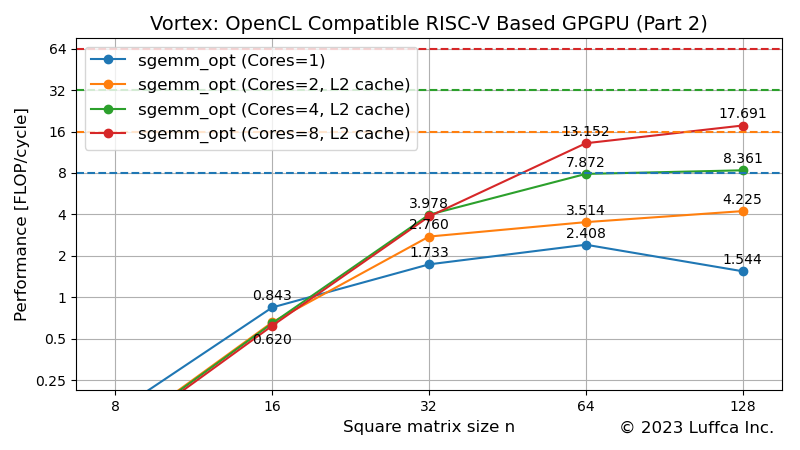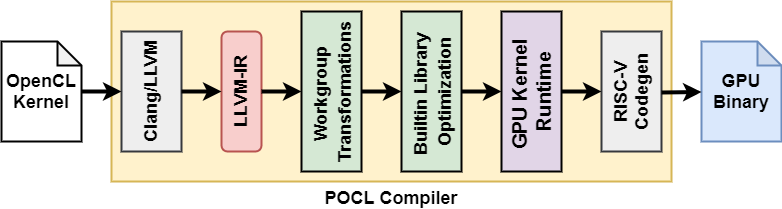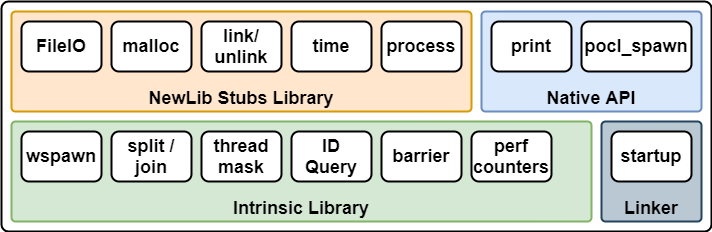# Vortex: OpenCL Compatible RISC-V Based GPGPU (Part 2)This article introduces the OpenCL support of Vortex, a RISC-V based open source GPGPU.

The related article Vortex: OpenCL Compatible RISC-V Based GPGPU (Part 1) introduces an overview of Vortex and how to run a test program using the Vortex simulator.

## Vortex

Vortex is a single instruction, multiple threads (SIMT) execution model GPGPU processor that adds custom instructions for GPGPU to RISC-V ISA. For an overview of Vortex, see the related article Part 1.

Vortex supports OpenCL 1.2. In this article, we’ll take a look at Vortex’s OpenCL support.

## Software Stack for OpenCL

Vortex uses the open source framework PoCL (Portable Computing Language) to support OpenCL. The software stack for OpenCL includes the PoCL compiler, PoCL runtime, and Vortex runtime.

The diagram below shows the flow of generating the OpenCL kernel binary from source code. The PoCL compiler uses Clang/LLVM internally.Vortex binary generation steps for OpenCL applications.

The PoCL compiler is configured to generate kernel programs targeting Vortex. Also, the PoCL runtime seems to have been modified to allow access to the Vortex driver.

The diagram below shows the configuration of the Vortex runtime. Newlib is used as the standard C library.Vortex Runtime

Vortex’s ISA is based on the RV32IMF, but adds custom instructions. The Intrinsic Library is implemented in the Vortex runtime to use the added custom instructions without modifying the existing Clang/LLVM.

## OpenCL Examples

There are OpenCL test programs in the `tests/opencl` directory of the Vortex repository. An OpenCL program is divided into host and device code, `main.[cc|cpp]` and `kernel.cl` respectively.

OpenCL speeds up by executing kernels in parallel on the device side. Let’s take a look at the code in `tests/opencl/sgemm` as a concrete example. Note that the code has been slightly modified to make the difference clearer.

The following shows the matmul function in `main.cc` running on the host.

```void matmul(const float* A,
const float* B,
float*       C,
int          N) {
for (int i = 0; i < N; ++i) {
for (int j = 0; j < N; ++j) {
float acc = 0.0f;
for (int k = 0; k < N; ++k) {
acc += A[i + k * N] * B[k + j * N];
}
C[i + j * N] = acc;
}
}
}
```

Below is the `kernel.cl` running on the device corresponding to the above functions.

```__kernel void sgemm(__global const float* A,
__global const float* B,
__global float*       C,
int                   N) {
const int i = get_global_id(0);
const int j = get_global_id(1);
float acc = 0.0f;
for (int k = 0; k < N; ++k) {
acc += A[i + k * N] * B[k + j * N];
}
C[i + j * N] = acc;
}
```

In `kernel.cl` the loop over i and j in the matmul function has been removed. Instead, each kernel is modified to compute only the (i, j) entry of matrix C given i and j. This is a mechanism that can speed up by executing kernels in parallel on the device side.

However, with parallelization alone, the latency for global memory becomes significant, so in practice, there are many examples of using private memory or local memory, which have low latency. However, there is Local memory issue #10 in the repository regarding the use of local memory in Vortex.

Let’s analyze the utilization of Vortex’s sgemm introduced in Part 1. The default number of threads is 4, so a quad-core configuration would have 16 threads running at the same time. Performance (FLOP/cycle) at matrix size 128 is 1.528 and its utilization is less than 5% (1.528/(2*16)*100).

## Running `sgemm_opt` on Vortex RTL Simulator

It is so inefficient that we tried to improve the efficiency of sgemm by using methods other than local memory.

Specifically, we used the Vortex register file, which is equivalent to OpenCL’s private memory, to block 4×4 and unroll the loop. Also, we added the FMA macro because the compiler converted `fmul.s` and `fadd.s` instead of FMA (`fmadd.s`).

The featured image shows the result of `sgemm_opt` improving efficiency. For a quad-core configuration, Performance (FLOPs/cycle) at matrix size 128 is 8.361, which is about a 5x improvement in performance due to optimization. And its utilization is about 26% (8.361/(2*16)*100). Even with the combination of FPGA implementation of 64-bit Rocket Chip and OpenBLAS, the utilization of SGEMM is less than 20%, so it has become a performance like GPGPU.

## Summary

This article introduced the OpenCL support of Vortex, a RISC-V based open source GPGPU. We also confirmed that the utilization of sgemm can be improved from less than 5% to about 26% by modifying the program.

Previous article

Next article